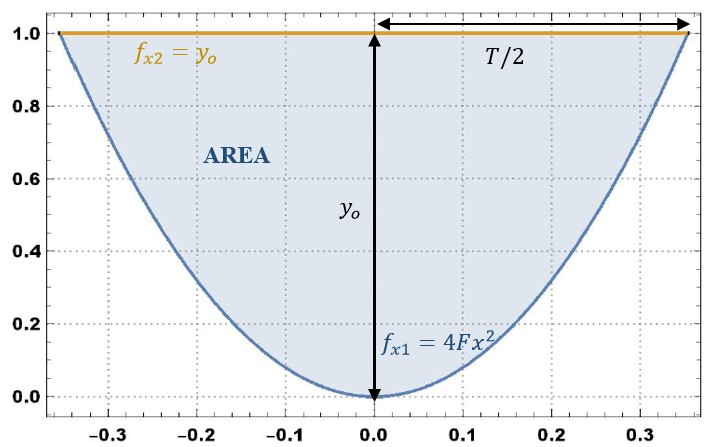Geometric properties of a parabolic section Jhonath W. Mejía and Víctor M. Ponce 210221

 ABSTRACT The purpose of this article is to formulate the equations for the calculation of the geometric properties of a parabolic section, in open-channel hydraulics. These equations are compared with those presented in Chapter 2 of Ven Te Chow's book "Open-channel Nydraulics" (1959).

1.   INTRODUCTION

The calculation of the geometric properties of a parabolic channel section is accomplished by applying normal and line integrals, making use of the parameters that characterize a parabola.

2.  GEOMETRIC PROPERTIES OF A PARABOLIC SECTION

The Manning equation is:

 A   Q  =  ____  R 2/3  So1/2              n (1)

in which Q = flow rate; A = flow area; R = hydraulic radius; So = bottom slope; and n = Manning's roughness. Therefore:

 Q n              A 2/3   _____  =  A  _____  So1/2            P 2/3 (2)

in which the section factor Fs is given as follows:

 A 5/3   Fs  =  ______             P 2/3 (3)

Therefore:

 A 5   Fs3  =  _____               P 2 (4)

The area and perimeter of a parabolic section corresponding to a flow depth yo and top width T are shown in Fig. 1.Fig. 1  Definition sketch of a parabolic section.

To calculate the area, the following integral is used:

 T/2   A  =  2 ∫  (fx2 - fx1) dx                    0 (5)

 T/2                          T/2   A  =  2 ∫  (yo) dx  -  2 ∫  (4Fx 2) dx                   0                              0 (6)

 x 3   A  =  2yo [ x ] 0T/2  -  8F [ ___ ] 0T/2                                           3 (7)

 FT 3   A  =  yoT -  _____                        3 (8)

in which 4F is a parameter of the parabola defined as follows:

 yo            4yo   4F  =  ______  =  _____             (T/2) 2        T 2 (9)

Therefore:

 yo   F  =  _____            T 2 (10)

Substituting Eq. 10 in Eq. 8:

 yo       T 3                    yoT   A  =  yoT  -   _____  _____  =  yoT  -  _____                         T 2       3                       3 (11)

Therefore:

 2yoT   A  =  ______               3 (12)

For the wetted perimeter, a line integral is used:

 T/2   P  =  2 ∫  [1 + (f 'x1) 2] 1/2 dx                    0 (13)

 T/2   P  =  2 ∫  [1 + (8F) 2x 2] 1/2 dx                    0 (14)

Equation 14 has the form of a known integral:    (a2+x2)1/2dx, and its solution is:

 x                                  sinh-1 (8Fx)   P  =  2 [ ___ [1 + (8F) 2x 2] 1/2 + _______________ ] 0T/2                  2                                        2 (8F) (15)

 T/2                                         sinh-1 (8FT/2)   P  =  2 { ______ [1 + (8F)2(T/2) 2] 1/2 + _________________ }                     2                                                  2(8F) (16)

Substituting Eq. 10 in Eq. 16:

 T/2                                                   sinh-1 (8(yo/T 2)(T/2))   P  =  2 { ______ [1 + (8(yo/T 2)) 2 (T/2) 2] 1/2 + ________________________ }                     2                                                            2(8(yo/T 2)) (17)

 T            16yo 2              T 2                   4yo   P  =  ____ ( 1 + ______ ) 1/2 + ______ sinh-1 ( ______ )              2              T 2                8yo                    T (18)

 T                  4yo                     T                     4yo   P  =  ____ [ ( 1 + ( ______ ) 2 ) 1/2 + ______ sinh-1 ( ______ ) ]              2                   T                      4yo                    T (19)

Equation 19 may be expressed in terms of logarithms as follows:

 sinh-1θ = ln [θ+ (1+θ 2) 1/2] (20)

Therefore:

 T                  4yo                   T            4yo                  4yo   P  =  _____ { [1 + ( _____ ) 2 ] 1/2 + _____ ln [ _____ + ( 1 + ( _____ ) 2 ) 1/2] }              2                   T                    4yo           T                     T (21)

Equation 21 is the same formula that appears in Table 2-1 of Ven Te Chow's 1959 book: “Open-channel Hydraulics”.

Figure 2 shows Eq. 19 in graphical form: P = f (T,yo), for 0 < T < 10; 0 < yo < 5:Fig. 2  Wetted perimeter versus flow depth and top width.

Equation 19 is used to construct the geometric properties of the parabola.

The free surface width T is:

 3A   T  =  _____            2yo (22)

 A   R  =  _____             P (23)

 2yoT                                                 ______                                                      3   R  =  ____________________________________________________              T                  4yo                    T                     4yo            ____ { [ 1 + ( ______ ) 2 ] 1/2 + ______ sinh-1 ( ______ ) }              2                   T                      4yo                   T (24)

 4yo                 4yo                     T                    4yo   R  =  _____ { [ 1 + ( ______ ) 2 ] 1/2 + ______ sinh-1 ( ______ ) } -1               3                    T                      4yo                  T (25)

The hydraulic depth D is:

 A          A   D  =  ____  =  ____             T         3A                        ____                        2yo (26)

Therefore:

 2yo   D  =  _____              3 (27)

Table 1 summarizes the properties of a parabolic section. The properties may be calculated online using Online geometric elements parabolic.

 Table 1.   Geometric properties of a parabolic section. Property Equation Formula Area Eq. 12 2yoT   A  =  ______           3 Wetted perimeter Eq. 19 T                 4yo                     T                     4yo   P  =  ___ { [ 1 + ( ______ ) 2 ] 1/2 + ______ sinh-1 ( ______ ) }    2                  T                     4yo                    T Topwidth Eq. 22 3A   T  =  ______            2yo Hydraulicradius Eq. 25 4yo                  4yo                    T                    4yo   R  =  _____ { [ 1 + ( ______ ) 2 ] 1/2 + ______ sinh-1 ( _____ ) } -1   3                    T                      4yo                   T Hydraulic depth Eq. 27 2yo   D  =  ______            3

APPENDIX I.   REFERENCES

Chow, V. T. 1959. Open Channel Hydraulics. McGraw-Hill, New York.

Leithold, L. 1998. Calculus, Seventh Edition, Oxford University Press - Harla México, S.A.

APPENDIX II.  NOTATION

The following notation is used in this document:

Q = flow rate;

n = Manning's coefficient;

So = bottom slope;

yo = (center) flow depth;

Fs = section factor;

A = flow area;

P = wetted perimeter;

T = top width;